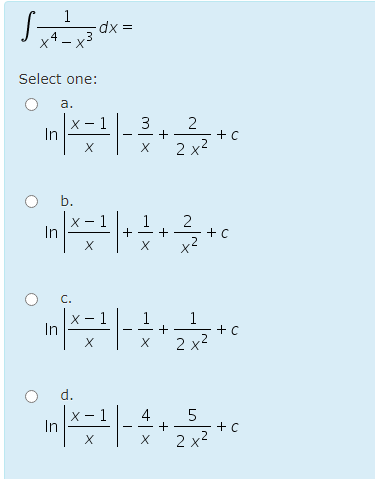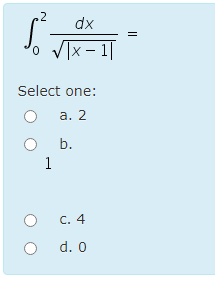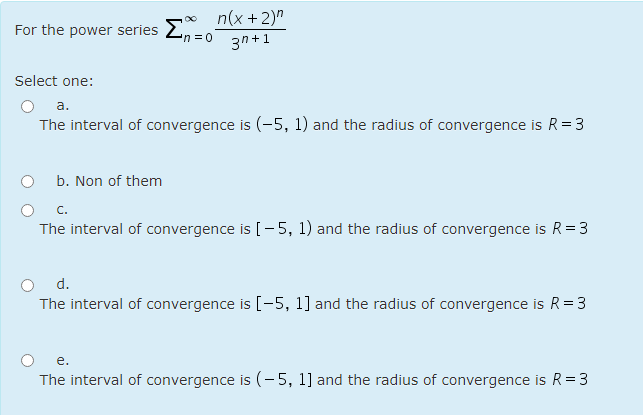### Create an Account

Already have account?

### Forgot Your Password ?

Home / Questions / -dx Select one: a. x-1 2 In 3 + X X 2x2 to b. - 1 In 1 + + X +C C. х 1 In 1 + х :+C х 2 x2...

# -dx Select one: a. x-1 2 In 3 + X X 2x2 to b. - 1 In 1 + + X +C C. х 1 In 1 + х :+C х 2 x2 d. 5 In 4 + X Х 2x3 to 2 \$ dx VIX - 11 Select one: : a. 2 b. 1 C. 4 d. O For the power series Σ 2 no n(x + 2)

-dx Select one: a. x-1 2 In 3 + X X 2x2 to b. - 1 In 1 + + X +C C. х 1 In 1 + х :+C х 2 x2 d. 5 In 4 + X Х 2x3 to
2 \$ dx VIX - 11 Select one: : a. 2 b. 1 C. 4 d. O
For the power series Σ 2 no n(x + 2) 3n+1 n = 0 Select one: a. The interval of convergence is (-5, 1) and the radius of convergence is R=3 b. Non of them C. The interval of convergence is [-5, 1) and the radius of convergence is R = 3 d. The interval of convergence is [-5, 1] and the radius of convergence is R = 3 e. The interval of convergence is (-5, 1] and the radius of convergence is R = 3Apr 24 2021 View more View Less

#### Answer (Solved)Subscribe To Get Solution